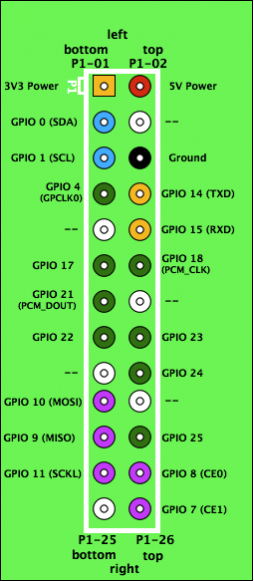# Cheat Sheet

## Basic GPIO Access

### SetupRaspberry Pi GPIO pin layout. Diagram from eLinux.

At the start of your file include:

``````import RPi.GPIO as GPIO
``````

This gives you access to the GPIO functions in the GPIO namespace.

Now set the mode to represent the numbering scheme you prefer.

``````GPIO.setmode(GPIO.BCM)
``````

sets the pin numbering of 1 to 26 as in illustration 1.

Setup the direction of a pin:

``````GPIO.setup(12,GPIO.OUT)
GPIO.setup(13,GPIO.IN,
pull_up_down=GPIO.PUD_UP)
``````

This code sets up pin 12 (GPIO 18) as an output and pin 13 (GPIO 21) as an input with a pull up resistor. `pull_up_down` can be `PUD_OFF`, `PUD_UP` or `PUD_DOWN`, (default `PUD_OFF`).

If you’re using our modified version of RPi.GPIO (you should be if using our image), then you have the extra feature of being able to turn on the alternate function for pin 4 (setting it up as a general purpose clock). Do this with:

``````GPIO.setup(4,GPIO.ALT0)
#set the clock to the frequency we want (in Hz), here we choose 64 Khz
#frequency should be between 4640 and 19000000
GPIO.setclock(4,64000)
#turn on the clock
GPIO.output(4,1)
#GPIO.output(4,0) if we want to turn it off again
#change the frequency to 500 KHz
GPIO.setclock(4,500000)

``````

With your pins set up in the proper direction:

``````input = GPIO.input(13)
GPIO.output(12,GPIO.HIGH)
#can be GPIO.HIGH, GPIO.LOW, 1, 0, True, False
#GPIO.output(12,GPIO.input(13)) would set 13 high whenever 12 is

``````

## Using I2C to control servos, LEDs and use PWM

### Setup

``````
import i2c
servos = i2c.I2C()
#leds = i2c.I2C(frequency=1000)
``````

The i2c class will by default set frequency to 50Hz to work well with servo pulses. If you don’t want to drive servos you can set it between 40 and 1000Hz to give more control and avoid flicker.

### Setting Speeds

``````
servos.setSpeeds(100,-100)
#this will set the left motor (on led0) to full speed, and right motor (led1) to full reverse
servos.setSpeeds(50,50)
servos.setPWM(15,4095)
#this will set an LED on led15 to full brightness (on for 4095 ticks)
servos.setPWM(14,2048)  #set led14 to half brightness

``````

## Choosing which programs run when the Pi boots

/etc/rc.local is a script on the Raspberry Pi which runs when Linux first boots. To edit it, you will need root privileges:

``````sudo nano /etc/rc.local
``````

If you want to run one of your Python scripts at start-up, add this to the end of rc.local:

``````python <yourscriptname>.py
``````

To stop a script running, either delete the line it is on, or comment it out (add a `#` at the beginning of the line).